ISEE Upper Level Math : Numbers and Operations

Example Questions

1 2 3 4 5 6 7 8 9 10 12 Next →

Example Question #111 : Numbers And Operations

Simplify: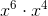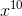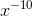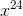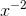Explanation:

When multiplying exponents, the exponents are added together.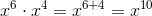Example Question #112 : Numbers And Operations

Simplify: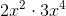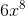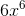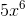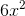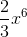Explanation:

When multiplying exponents, the exponents are added together.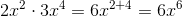Example Question #113 : Numbers And Operations

Simplify the expression: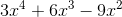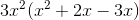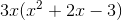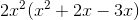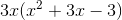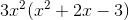Explanation:

To simplify this problem we need to factor out a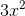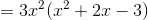We can do this because multiplying exponents is the same as adding them. Therefore,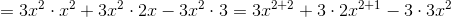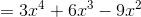Example Question #114 : Numbers And Operations

Solve: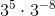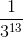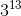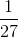Explanation:

It is not necessary to evaluate both terms and multiply.

According to the rules of exponents, when we have the same bases raised to some power that are multiplied with each other, we can add the powers.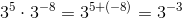This term can be rewritten as a fraction.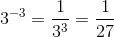The answer is:Example Question #115 : Numbers And Operations

Which of the following is equivalent to the expression below?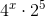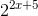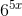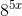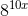Explanation:

When exponents are multiplied by one another, and the base is the same, the exponents can be added together.

The first step is to try to create a common base.Given that the square of 2 is for, the expression can be rewritten as: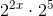Example Question #116 : Numbers And Operations

Simplify: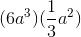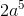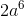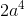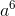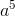Explanation:

Based on the product rule for exponents in order to multiply two exponential terms with the same base, add their exponents: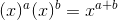So we can write: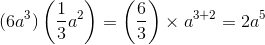1 2 3 4 5 6 7 8 9 10 12 Next →